Home > ACC7 > Chapter cc29 > Lesson cc29.2.3 > Problem9-80

9-80.
1. Simplify each of the following expressions. Homework Help ✎

1. 82 + 72

2. 72 · 7 · 7

3.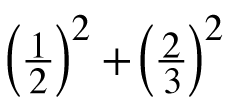4. (22 + 6)2

5. −4 · 32 − 2

6. x x

7. 3 · 3 ÷ 3

8. 4x + x − 2x

9. −2 · 4 ÷ 4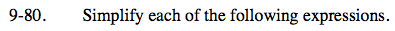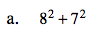Use the Order of Operations.

64 + 49

113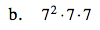How can you combine these?

74 = 2401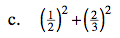Use the same method as part (a).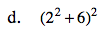(4 + 6)2

(10)2

100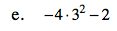Use the Order of Operations.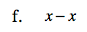What do you get when you subtract any number from itself?

0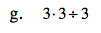Start from the left and work your way to the right.

3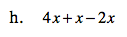Combine like terms.

3x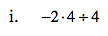See part (g).